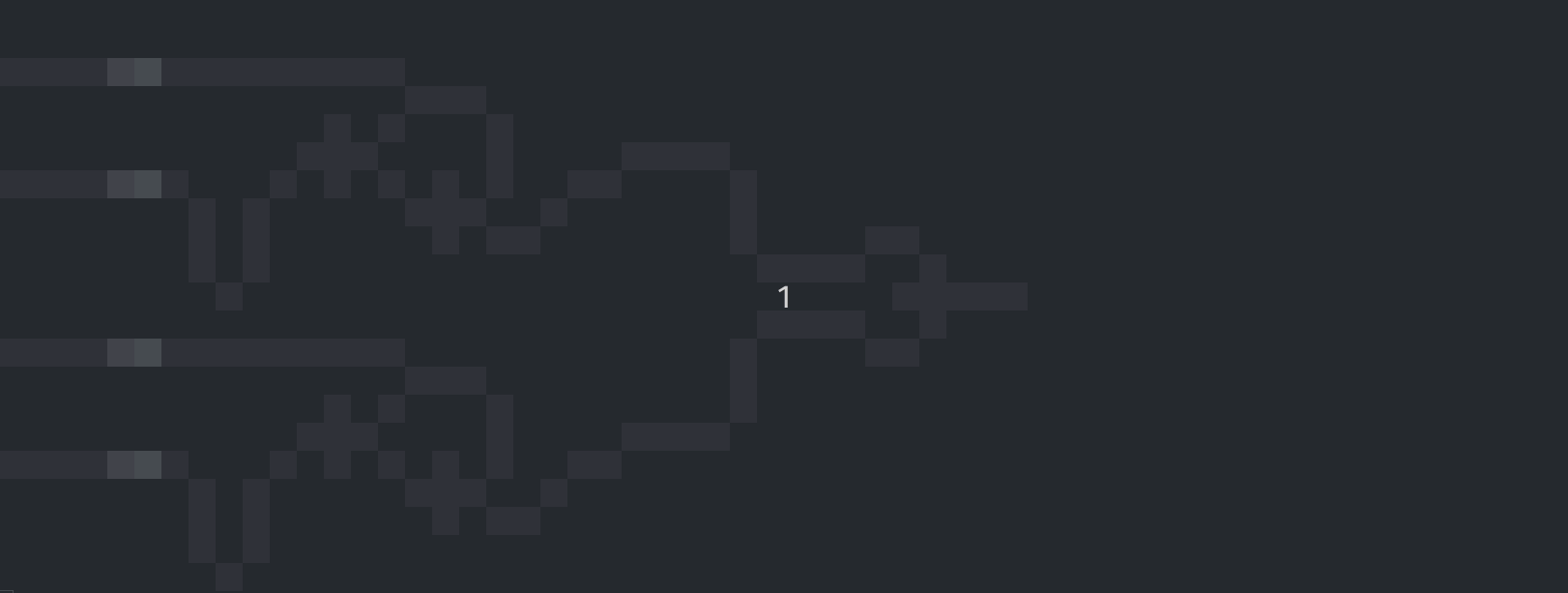# Cellular Automaton in Haskell (II). WireWorld

Learning Haskell, projects such as Conway's Game of Life and WireWorld are valuable to me. They are narrow, include interesting parts, and don't take too much time to implement. Most of all, they're fascinating 'games' in themselves.

When making WireWorld we can reuse large parts (with some trivial changes) of the UI made for the implementation of Conway's Game of Life, described in the previous post.These are the rules for WireWorld:

A cell has four possible states:

• empty (1),
• electron tail (3),
• conductor (4).

Each cell evolves from one generation to the next and depending on the game circumstances its state may change. Change occurs according to his schedule:

• empty → empty,
• electron head → electron tail,
• electron tail → conductor,
• conductor → electron head if exactly one or two of the neighbouring cells are electron heads, otherwise remains conductor.

In fact, with some trivial changes, we can reuse pattern formatting as well. I store 'patterns' in a similar fashion using a text file. This is a logical gate for OR I've borrowed from Wolfram:

``````.......**.....................
h******..*....................
........**********************
h******..*....................
.......**.....................
``````

If you compare with my implementation of Conway's Game of Life the only important thing changed is name of the function determining a cell state, and the conditions (since WireWorld have four possible states and Conway's Game of Life only have two):

``````stateEMPTY     = 0
stateTAIL      = 2
stateCONDUCTOR = 3

main ∷ IO ()
main = do
get (prepareData \$ lines rawData)

representation ∷ State → String
representation node
| node == stateHEAD      = "██"
| node == stateTAIL      = "▓▓"
| node == stateCONDUCTOR = "░░"
| otherwise              = "  "

putNode ∷ Node → IO ()
putNode node
| fst (fst node) == 0 = putStr \$ "\n" ++ representation (snd node)
| otherwise           = putStr \$ representation (snd node)

clearScreen ∷ IO ()
clearScreen = putStr "\ESC[2J"

get ∷ Game → IO ()
get game = do
sequence_ [putNode node | node ← game]
clearScreen
get (nextState game)

nodeState ∷ Char → Integer
nodeState char
| char == '.' = stateEMPTY
| char == 'h' = stateHEAD
| char == '*' = stateCONDUCTOR
| otherwise   = stateTAIL

makeRow ∷ String → Int → [Node]
makeRow row y =
[((x,y), nodeState \$ row !! x) | x ← [0..length row - 1]]

prepareData ∷ [String] → Game
prepareData rawData =
concat [ makeRow (rawData !! y) y | y ← [0..length rawData - 1]]``````

As for the logic, we use similar procedures, only slightly refactoring my implementation of the logic for Conway's Game of Life:

``````directions ∷ [Point]
directions = [(0,-1), (1,-1), (1,0), (1,1), (0,1), (-1,1), (-1,0), (-1,-1)]

getNode ∷ Point → Game → Maybe Node
getNode pos [] = Nothing
getNode pos (((x,y), status) : rest)
| pos == (x,y) = Just ((x,y), status)
| otherwise = getNode pos rest

isHead ∷ Maybe Node → Integer
isHead node = case node of
Just node → if snd node == stateHEAD
else stateCONDUCTOR
Nothing   → stateCONDUCTOR

checkNeighbors ∷ Node → Game → [Point] → Integer → Integer
checkNeighbors ((x,y), nodeState) game dirs isAny
| null dirs          = stateCONDUCTOR
| otherwise          = checkNeighbors
((x,y), nodeState)
game
(tail dirs)